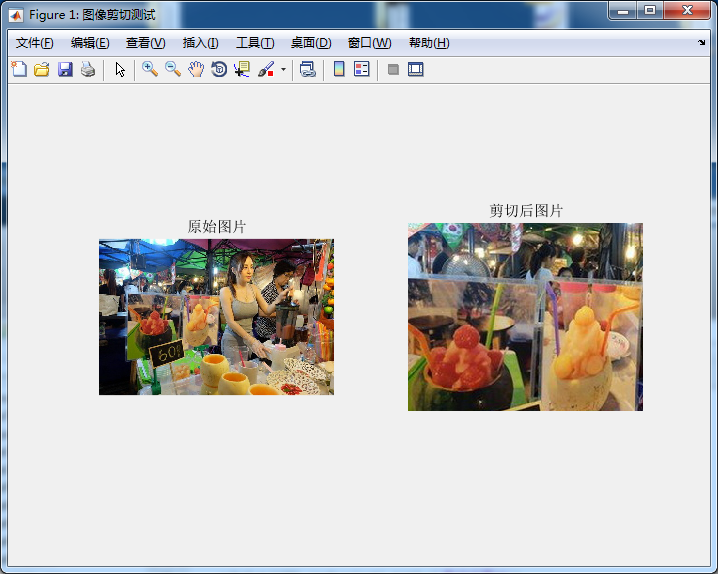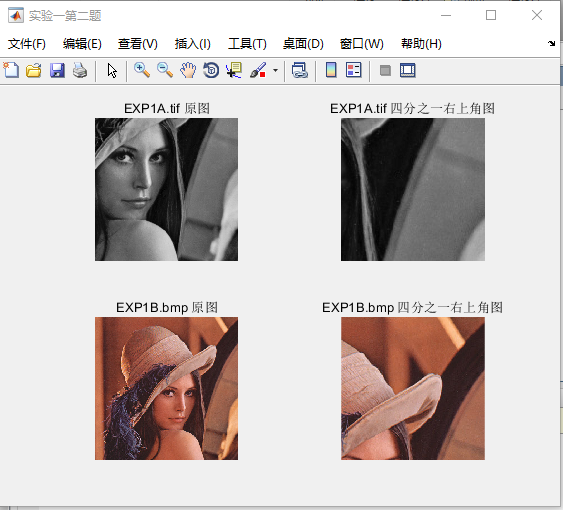• ## matlab图像剪切

千次阅读 2016-12-03 19:02:59
学习图像剪切matlab源码 function [imout] = ImageCrop( imin,x,y,width,height ) %UNTITLED13 此处显示有关此函数的摘要 % 此处显示详细说明 [m,n,g] = size(imin); imin = im2double...
趁热打铁！
学习图像剪切。

matlab源码
function [imout] = ImageCrop( imin,x,y,width,height )
%UNTITLED13 此处显示有关此函数的摘要
%   此处显示详细说明
[m,n,g] = size(imin);
imin = im2double(imin);
imout = zeros(width,height,g);
for i= 1:width
for j = 1:height
if (i+x <= m && j+y <=n)
imout(i,j,:) = imin(i+x,j+y,:);
end
end
end
end

图像剪切函数测试代码

clc;
clear;
figure('name','图像剪切测试');
subplot(121);imshow(i);title('原始图片');
out = ImageCrop(i,100,100,200,250);
subplot(122);imshow(out);title('剪切后图片');展开全文• Matlab图像剪切，超出图像区域指定颜色填充，Matlab自带图像剪切（裁剪）函数，即imcrop函数，但该函数对于超出图像边界区域是停止裁剪的，即对超出图像边界的部分不作裁剪处理。正常情况下，imcrop函数足够使用了。...
Matlab图像剪切，超出图像区域指定颜色填充  【尊重原创，转载请注明出处】http://blog.csdn.net/guyuealian/article/details/77507969   Matlab自带图像剪切（裁剪）函数，即imcrop函数，但该函数对于超出图像边界区域是停止裁剪的，即对超出图像边界的部分不作裁剪处理。正常情况下，imcrop函数足够使用了。但有时，我们希望对于超出图像边界的区域，可以指定颜色填充，以便我们可以观察裁剪情况。   若想在OpenCV中实现图像剪切，对超出图像区域指定颜色填充，可参考本人的博客：http://blog.csdn.net/guyuealian/article/details/77486096   本人实现方法如下：function destImg=ImageCropPadding(gray_img,rect,paddingValue)
% 输入参数：gray_img：必须输入灰度图像，暂时不支持RGB图像；
%              rect：指定剪切区域，类似于Matlab的imcrop函数用法
%% For Example:
%  grabImage=rgb2gray(SrcImage);
%  figure,imshow(grabImage),title('grabImage');
%  rect=[-50,-50,500,500];
%  figure,imshow(destImg),title('destImg');
%%
x=rect(1);
y=rect(2);
w=rect(3);
h=rect(4);
crop_x1 = max(1, x);
crop_y1 = max(1, y);
[rows,cols]=size(gray_img);
crop_x2 = min(cols, x + w);
crop_y2 = min(rows, y + h);
roi_img = gray_img(crop_y1:crop_y2,crop_x1:crop_x2);
x1 = crop_x1 - x+1;
y1 = crop_y1 - y+1;
x2 = crop_x2 - x+1;
y2 = crop_y2 - y+1;
% I(y1:y2, x1:x2 ) = I(y1:y2 , x1:x2) + roi_img;
destImg(y1:y2, x1:x2 ) =  roi_img;
end   调用方法：SrcImage=imread('images/liuyifei.jpg');
grabImage=rgb2gray(SrcImage);
figure,imshow(grabImage),title('grabImage');
rect=[-50,-50,500,500];
figure,imshow(destImg),title('destImg');   运行效果：

展开全文• matlab按步长剪切图片：clear;close all;%% settingsfolder = 'E:\train\';savepath = 'F:\matlab\';size_input = 60;stride = 40;%% initializationdata = zeros(size_input, size_input, 1, 1);count = 0;%% ...
matlab按步长剪切图片：clear;close all;%% settingsfolder = 'E:\train\';savepath = 'F:\matlab\';size_input = 60;stride = 40;%% initializationdata = zeros(size_input, size_input, 1, 1);count = 0;%% generate datafilepaths = dir(fullfile(folder,'*.bmp'));for i = 1 : length(filepaths)image = imread(fullfile(folder,filepaths(i).name));image = rgb2ycbcr(image);image = im2double(image(:, :, 1));[hei,wid] = size(image);for x = 1 : stride : hei-size_input+1for y = 1 :stride : wid-size_input+1count=count+1;subim_input = image(x : x+size_input-1, y : y+size_input-1);imwrite(subim_input,strcat(savepath,num2str(count),'.bmp'),'bmp');endendend重命名：clear;close all; %% settings folder = 'D:\SYM\Caffe\caffe-master\mypro\GAN\data\train\'; savepath = 'F:\matlab\'; %% initialization count = 2386; %% generate data filepaths = dir(fullfile(folder,'*.jpg'));    for i = 1 : length(filepaths)      image = imread(fullfile(folder,filepaths(i).name));     count=count+1;             imwrite(image,strcat(savepath,num2str(count),'.jpg'),'jpg'); end
展开全文• 读入实验图像EXP1A.tif和EXP1B.bmp，分别剪切出上述图像右上角四分之一的内容，并要求在同一个图形界面上显示。 要求： 在同一个图形界面上，于第1行第1列位置上显示图像EXP1A.tif的原始内容，于第1行第2列位置上...
读入实验图像EXP1A.tif和EXP1B.bmp，分别剪切出上述图像右上角四分之一的内容，并要求在同一个图形界面上显示。

要求：

在同一个图形界面上，于第1行第1列位置上显示图像EXP1A.tif的原始内容，于第1行第2列位置上显示EXP1A.tif右上角四分之一的内容，于第2行第1列位置上显示图像EXP1B.bmp的原始内容，于第2行第2列位置上显示EXP1B.bmp右上角四分之一的内容。

实现思路：

2.使用size函数读取图像的行数r和列数c，将两幅图像的行数和列数分别除以2：r/2、c/2，因为运算结果可能不是整数，所以需要使用ceil函数来对结果进行取整得到二分之一行数half_x和列数half_y

3.分别取两幅图像的1至half_x行和half_y至最后一行

4.按题目要求显示图像

实现效果：实现源代码：

clc;
%读取图像

[ar,ac,ak] = size(img_a);%取图像的行数r和列数c
%取图像右上角四分之一
half_ax = ceil(ar/2);%ceil是对r/2的结果取整(向正无穷方向)
half_ay = ceil(ac/2);
img_a1 = img_a(1:half_ax,half_ay:end);

[br,bc,bk] = size(img_b);%取图像的行数r和列数c
%取图像右上角四分之一
half_bx = ceil(br/2);%ceil是对r/2的结果取整(向正无穷方向)
half_by = ceil(bc/2);
img_b1 = img_b(1:half_bx,half_by:end,:);

%显示图像
figure('NumberTitle', 'off', 'Name', '实验一第二题');
subplot(2,2,1);
imshow(img_a);
title('EXP1A.tif 原图');

subplot(2,2,2);
imshow(img_a1);
title('EXP1A.tif 四分之一右上角图');

subplot(2,2,3);
imshow(img_b);
title('EXP1B.bmp 原图');

subplot(2,2,4);
imshow(img_b1);
title('EXP1B.bmp 四分之一右上角图');



展开全文image
• Matlab GUI 实现图像截取，可以用鼠标控制矩形框的大小。存储图像块，并且发布GU程序。
• 【实例简介】剪切波变换MATLAB实现代码，包含2D及3D图片的，还有对应的反变换代码【实例截图】【核心代码】ShearletTransform_Matlab└── 2D├── dfilters.m├── dmaxflat.m├── MakeONFilter.m├── ...
• Matlab GUI 实现图像截取，可以用鼠标控制矩形框的大小。存储图像块，并且发布GU程序。 文件： https://590m.com/f/25127180-485265234-bf6a0d （访问密码：551685） 以下内容无关： ------------------------------...
• MATLAB图像批量剪切Github: loper-eswai/MagicLab 问题描述源文件夹下有若干子文件夹，包含不同类别的图片文件。现要求将每个图片剪切成相同大小，保存在目标文件夹。解决方案参数设置： - src_dir： 源文件夹 ...
• 【题目】剪切波的一些知识(附python及matlab实现剪切波变换的代码）   概述  本文是阅读论文《基于剪切波变换的人脸表情识别》后，对于其中有关剪切波内容的一些笔记。  剪切波是一种多尺度几何分析工具，...代码实现 python
• matlab按步长剪切图片： clear;close all; %% settings folder = 'E:\train\'; savepath = 'F:\matlab\'; size_input = 60; stride = 40; %% initialization data = zeros(size_input, size_input, 1, 1); count =
• matlab剪切图片代码场景完成 Matlab计算摄影 使用21个matlab文件 sceneCompletion_starter.m make.m ----来自Miki Rubinstein applyMask.m gistCompute.m gistComputeInput.m imresizecrop.m ----来自奥利瓦（Oliva...
• 图片的旋转： imrotate(image,90);图片剪切： imcrop(image,[10 10 50 60 ]);image
• 用于将巨幅图片剪切为规定大小的matlab代码，图像剪切、机器学习、深度学习、图像目标识别和检测。
• matlab剪切图片代码验证码识别 该存储库主要是关于自动识别验证码的。 一种称为“滴水算法”的方法用于将图片切成多个部分，便于后续的识别操作。 介绍 ./cut_algorithm ：主要是用matlab语言编程的cut算法。 ./cut_...
• matlab剪切图片代码SFND_radar_target 雷达目标产生与探测项目 依存关系 Matlab> = R2018b（运行程序的Matlab版本） 带有matplotlib和numpy的Python> = 3.6（运行程序的Python版本） 已在Ubuntu 18.04下测试。 运行...
• 图像剪切（crop）matlab %imcrop I=imread('lena.jfif'); I1=imcrop(I,[0,0, 150,150]); subplot(121) imshow(I); subplot(122) imshow(I1); 代表剪切到的图像为（0,0）与（150，150）所围成的区域
• matlab剪切图片代码ST匹配 MATLAB实现地图匹配问题的算法。 论文摘要 地图匹配是将观察到的用户位置序列与数字地图上的道路网络对齐的过程。 这是许多应用程序的基本预处理步骤，例如运动对象管理，交通流分析和行车...
• matlab程序批量根据鼠标点两下选中图片矩形区域剪切保存 可直接运行
• matlab剪切图片代码导航 概述 该matlab脚本使用来自网络摄像头的实时供稿来查找目标的中心，然后确定与目标的距离，并播放基于距中心的距离而频率增加的音频。 该项目的最初目的是制造一种盲人可以使用的步枪。 它被...
• matlab剪切图片代码算法反分化：以PageRank，最小切割和流量为例的案例研究 大卫·格莱希（David F. Gleich） 迈克尔·W·马洪尼 内容 这些是ICML 2014论文随附的计算代码和数据 David F. Gleich and Michael ...gui
• 1.编写 M 文件。  用过 Matlab 的人应该都接触过 M 文件
• 剪切波变换MATLAB实现代码，包含2D及3D图片的，还有对应的反变换代码
• function[]=cropG()% 从路径输入源图片---利用gt.txt剪切---输出到目标文件 ObjDir = 'G:\matlab_code\ori_image\Biker\Biker\img\';%需要剪切图片文件夹路径 OutputDir = 'G:\matlab_code\image\'; %输出文件的...
• 图像剪切的代码matlab 肺血管分割使用图切 使用以下链接准备图切优化器： 解压缩“ GCmex1.9.tar.gz”，并按照相应的说明编译图形切割的mex文件。 在Matlab中运行Demo.m文件 如果您使用该软件，则应参考以下文章： @......

# matlab图像剪切matlab 订阅